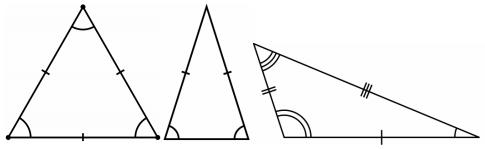favorite Нам необхідно трохи Вашої допомоги щоб сайт продовжував працювати, натисніть на банер щоб дізнатись більше.
Задачі

# Triangle

A triangle is one of the basic shapes of geometry: a polygon with three corners or vertices and three sides or edges which are line segments.

Triangles can be classified according to the relative lengths of their sides:

• In an equilateral triangle all sides have the same length. An equilateral triangle is also a regular polygon with all angles measuring 60°.
• In an isosceles triangle, two sides are equal in length. An isosceles triangle also has two angles of the same measure; namely, the angles opposite to the two sides of the same length; this fact is the content of the Isosceles triangle theorem.
• In a scalene triangle, all sides are unequal. The three angles are also all different in measure. Some (but not all) scalene triangles are also right triangles.Input

The first line of input contains an integer (1T100), the number of test cases. T test data sets follow, each data set consists of 3 integers A, B and C, where (1A, B, C1000000) the triangle side lengths.

Output

For each test case, print "equilateral", "isosceles" or "scalene" describing the triangle type. If the input doesn’t create a valid triangle output "invalid!". Follow the output format below.

Ліміт часу 1 секунда
Ліміт використання пам'яті 64 MiB
Вхідні дані #1
2
3 3 4
6 4 2

Вихідні дані #1
Case #1: isosceles
Case #2: invalid!

Джерело The Third Lebanese Collegiate Programming Contest